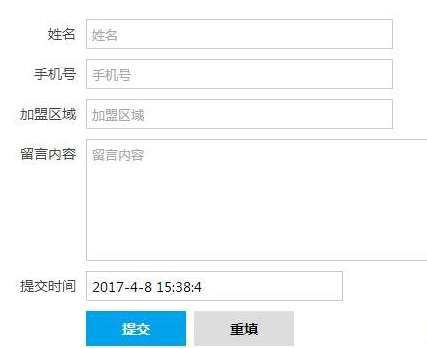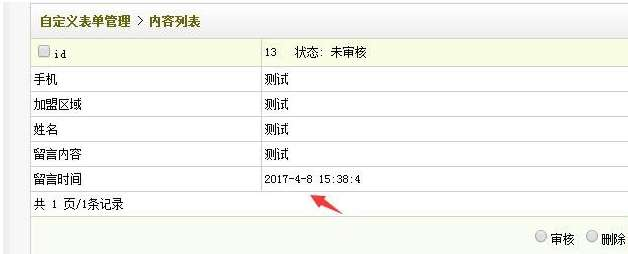# 织梦dedecms怎么给自定义表单添加提交时间

##### 92建站   织梦CMS   2021-04-20   收藏本文

<form action="/plus/diy.php" enctype="multipart/form-data" method="post" name="form" onsubmit="return CheckForm();">

<input type="hidden" name="action" value="post" />

<input type="hidden" name="diyid" value="1" />

<input type="hidden" name="do" value="2" />

<input name="mytime" value="" type="text"  id="mytime"  /> <!-- 如不需要在前台显示的话可以修改type="hidden" -->

<script type="text/javascript">

var nowDate = new Date(); var str = nowDate.getFullYear()+"-"+(nowDate.getMonth() + 1)+"-"+nowDate.getDate()+ " "+nowDate.getHours()+":"+nowDate.getMinutes()+":"+nowDate.getSeconds();

document.getElementById("mytime").value=str;

}

</script>

<input type="hidden" name="dede_fields" value="mytime,textchar" />

<input type="hidden" name="dede_fieldshash" value="849a871768b5942ee259e8f7af736194" />

<label><input class="btn_tj" type="submit" name="Submit" value=" 提交" /></label> </form>(此图片来源于网络，如有侵权，请联系删除! )

<input name="mytime" value="" type="text"  id="mytime"  /><!-- 如不需要在前台显示的话可以修改type="hidden" -->(此图片来源于网络，如有侵权，请联系删除! )